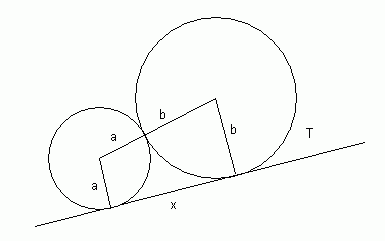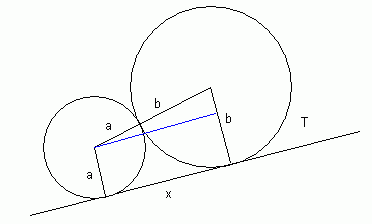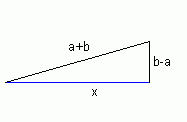SEARCH HOMEMath Central Quandaries & QueriesQuestion from erson, a student: this is the last..... please help me i humbly request.....if possible....may i get the answer tomorrow... because i'll be needing this the day after tomorrow... find the length of the tangent segment AB to two circles whose radii are a and b respectively, when the circles touch each other. the illustration looks like this...hope you'll understand... there are 2 circles - one is big one is small. they touch each other. and there is this irregular 4 sided polygon that connects them...there is a line that connects them from their center point and another from the tip of the circles...and that's it...i cannot explain very well please bear with meI think you are looking at this diagram:Remember that radii meet points of tangency at right angles, so that means the two radii drawn are parallel to each other. Now if I draw a blue line parallel to the tangent line here:Then I have created a rectangle. Therefore the length of the blue line is the same as the length x you are trying to find. As well, you know the lengths of two of the sides of the triangle you see there and can show that it is a right triangle:Solve for x.

Cheers,
Stephen La Rocque.Math Central is supported by the University of Regina and The Pacific Institute for the Mathematical Sciences.